Dataset - Tencent ML-Images 腾讯多标签数据集 - AIUAI

## 1. 综述

 - 构建了大规模图像数据集 - Tencent ML-Images，其包含 18M 张图片和 11K 个类别.

 - 基于 Tencent ML-Images 数据集，训练多输出的 ResNet-101 模型. 采用大规模分布式深度框架，训练了 60 个 epochs，耗时 90 hours.

 - 提供的模型断点(checkpoints)，具有很好的视觉表示能力；其在三个任务场景的迁移学习中被验证，即：ImageNet 和 Caltech-256 的单标签图像分类；PASCAL VOC 2007 的目标检测；PASCAL VOC 2012 的语义分割.

 - 为什么需要大规模图像数据集？

 - 为什么需要多标签图像数据集？

• 图片并非从 Google 或 Flicker 上收集新的图片，而是基于 OpenImages 和 ImageNet 数据集.
• 主要合并了两个数据集的类别标注词汇为统一标注词汇，移除了很稀少和无用的类别标注及对应的图片；然后进一步根据 WordNet 构建的标注词汇的语义层次词汇.
• 基于 OpenImages 和 ImageNet 的原始标注标签，派生了类别的共生性(co-occurrence)词汇，然后与语义层次词汇一起作为增强的标注标签.

 - 为了缓解类别不均衡的问题，设计了新的损失函数，其考虑了加权交叉熵(weighted cross entropy)，训练过程中损失权重的自适应，和每个 mini-batch 中负训练图片的下采样.

 - 为了加速训练过程，采用了大规模分布式深度学习框架，如 TFplus，采用了 Message Passing Interface (MPI) 和 NVIDIA Collective Communications Library (NCCL). 128 GPUs 训练 60 个 epochs，耗时 90 hours.

## 2. Tencent ML-Images 数据集收集

### 2.1. 图像源和类别词汇

 - 首先，从 ImageNet-11K 提取图像 URLs.

ImageNet-11K 是 MXNet 收集的 ImageNet 数据集的一个子集，其共包含了 11797630 张训练图像，包含了 11221 个标注类别. 不过，这 11221 个类别中的 1989 个类别标注是视觉非常抽象的，如 eventsummer 等. 这些抽象标签的标注可能不会对图像的视觉表示有帮助.

 - 然后，移除抽象标注的标签类别，以及对应的图像.

 - 接着，根据类别间的语义关系，根据 ImageNet 的标注词汇，新增了 800 个更精细的类别.

 - 对于 OpenImages 数据集，采用每个类别标准，过滤数据集中的所有图片.

 - 合并 ImageNet 和 OpenImages 中选择的 URLs 和类别词汇，构成了 Tencent ML-Images 的 URLs 和标签词汇.

### 2.2. 图像的类别标签增强

ImageNet-11K 的每张图片只标注了一个标签. Tencent ML 数据集通过利用类别间的语义层次和共生性对图片的标注标签进行增强.

 - 首先，根据语义层次，原始的单个标签的所有父类别(ancestor categories) 均被标注为该图片的标签.

 - 然后，计算 ImageNet-11K 类别和 OpenImages 类别的共生矩阵 CO. 即：

### 2.3. 数据集统计

# 训练图片# 验证图片# 类别# 训练类别# 平均每张图片的标签数# 平均每个类别的图片数
17,609,75288,73911,16610,50581447.2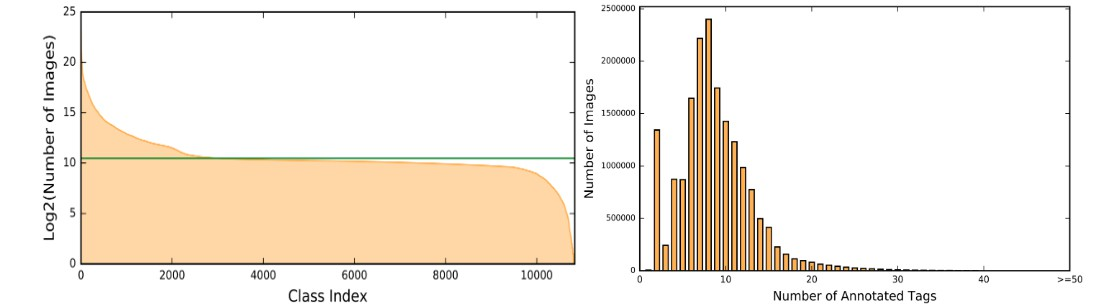## 3. 基于 Tencent ML-Images 数据集的视觉表示学习

### 3.1. 训练多输出的 ResNet-101 模型

#### 3.1.1. 模型和损失函数

$$\mathcal{L}_{\mathbf{w}}(\mathbf{x}_i, \mathbf{y}_i) = \frac{1}{m} \sum_{j}^{m} r_t^j [- \eta y_{ij}log(p_{ij}) - (1-y_{ij}log(1 - p_{ij}))]$$

$p_{ij} = f_{\mathbf{w}} (\mathbf{w}_i, j) \in [0, 1]$ 为关于类别 j 的后验概率，$\mathbf{w}$ 为待训练参数.

$\mathbf{y}_i = [y_{i1}, ..., y_{ij}, ..., y_{im}] \in \lbrace 0, 1 \rbrace^m$ 表示图像 $\mathbf{x}_i$ 的标注的标签向量.

$r_t^j = 0.9^{t-1}$ 表示在训练过程中的自适应权重. 对于类别 j，如果一个 mini-batch 内所有的训练图像都是负样本，则记录其状态为 0；如果至少有一张训练图片是正样本，则记录其状态为 1. 因此，可以得到一个状态向量：$(..., 0, 1, 1, 1, 0, 0, 1, 0, ...)$. 则，t 的定义为：如果当前 mini-batch 的状态与最后一个 mini-batch 不同，如，01和10，则 t=1; 如果当前 mini-batch 的状态与最后一个 mini-batch 的状态相同，则，t = t+1.

With $r_t^j$ , if the parameters corresponding to category j are positively or negatively updated in sequential mini-batches, the weight of the corresponding loss function is decayed. It will alleviatet he imbalance among frequent and rare categories.

#### 3.1.2. 图像预处理

 - 随机在图像中裁剪一个框(bounding box)，并保证框内图像的面积在整张图像的 [0.5, 1.0] 范围，图像的 width 和 height 比例在 [3/4, 4/3] 范围.

 - 将裁剪的图像尺寸调整为 224x224.

 - 以 0.5 的概率随机水平翻转.

 - 以 0.25 的概率在 [-45, 45] 的范围旋转图像.

 - 以 0.5 的概率颜色变换.

 - 将图像像素值范围由 [0, 1] 变换到 [-1, 1].

#### 3.1.3. 训练算法和参数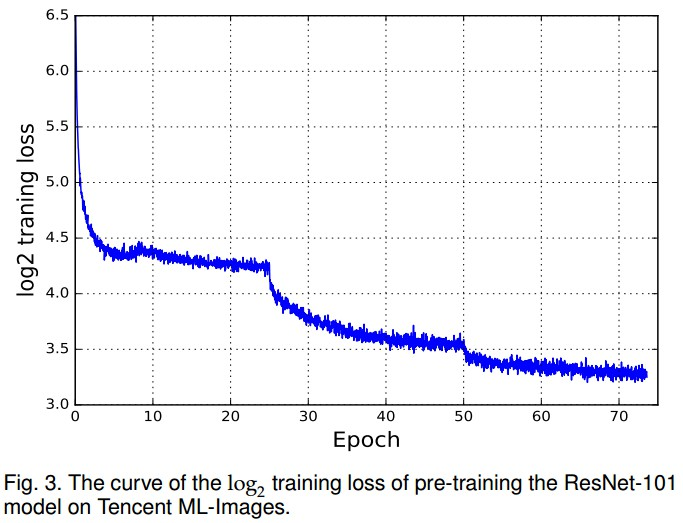#### 3.1.4. 分布式训练

ResNet-101 模型，11K outputs，18M 张训练图片，单机训练需要 12 天左右.

Tencent AI Lab 采用了大规模分布式深度学习框架，TFplus. 基于 TensorFlow. 替换了原始基于 MPI 的 gRPC 实现为 NCCL.

NCCL provides a highly optimized version of routines, such as all-gather, all-reduce, broadcast, reduce, reduce-scatter, especially the integrated bandwidth-optimal ring all-reduce algorithm, to achieve high bandwidth over PCIe on NVIDIA GPU.

### 3.2. 评价标准和结果

$$P_k = \frac{1}{n} \sum_i^n P_{i, k} = \frac{1}{n} \sum_i^n \frac{\mathbf{y}_i * \hat{\mathbf{y}}_i^k}{k}$$

$$R_k = \frac{1}{n} \sum_i^n R_{i, k} = \frac{1}{n} \sum_i^n \frac{\mathbf{y}_i * \hat{\mathbf{y}}_i^k}{\mathbf{1}^T * \mathbf{y}_i}$$

$$F1_k = \frac{1}{n} \sum_i^n \frac{2P_{i,k} * R_{i, k}}{P_{i, k} + R_{i, k}}$$

Top-5 和 Top-10 预测结果如：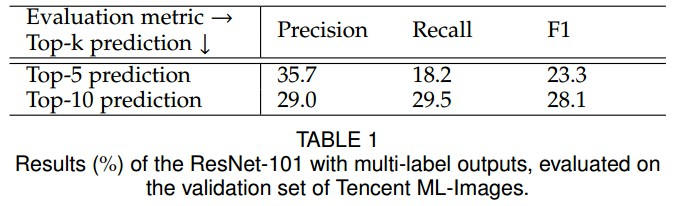## 4. 多标签模型的迁移学习

### 4.1. 迁移到ImageNet的单标签图像分类任务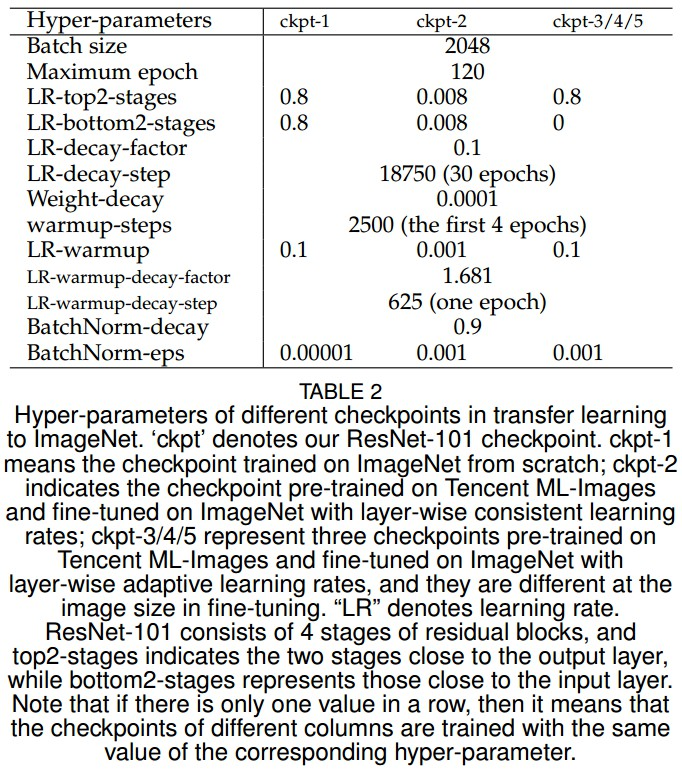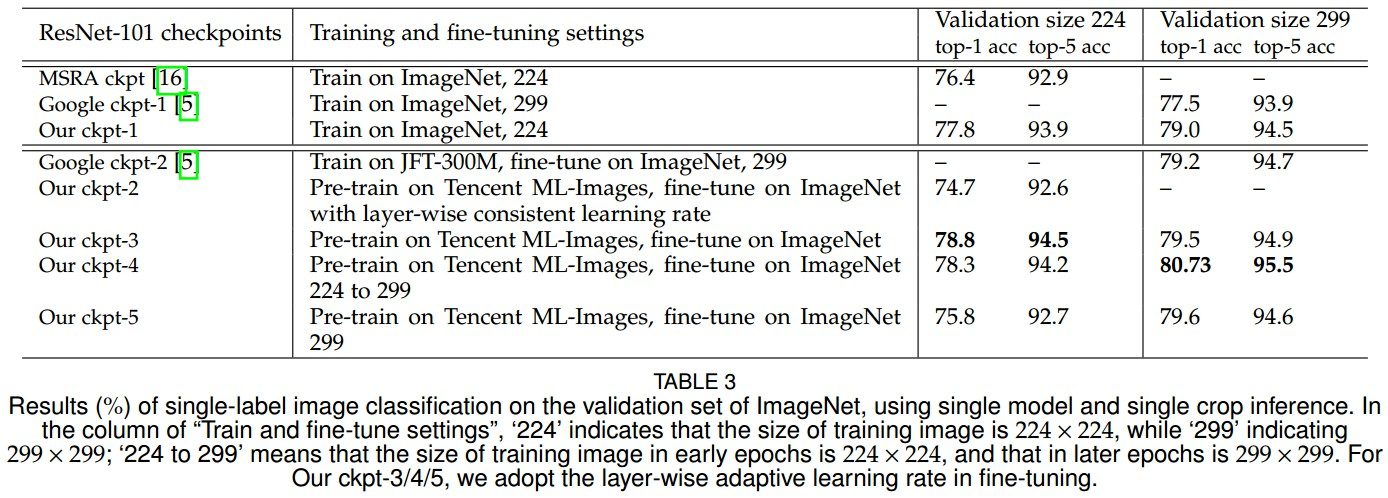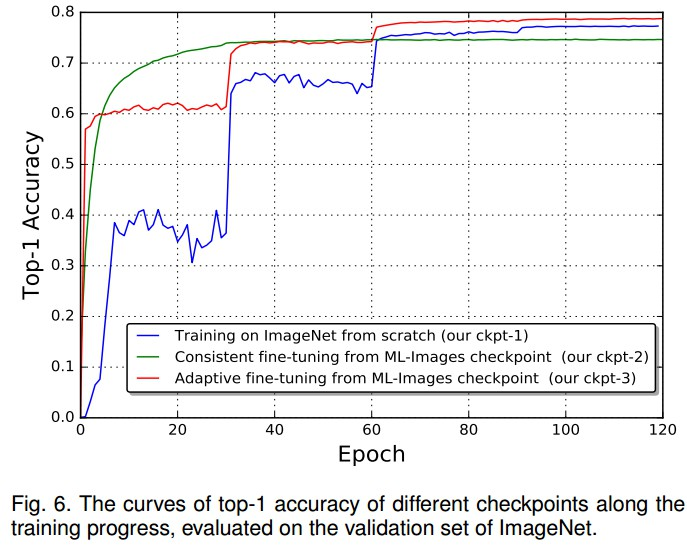### 4.2. 迁移学习到 Caltech-256 单标签图像分类任务

Caltech-256，小规模单标签图像数据集，共 30607 张图片，256 个类别.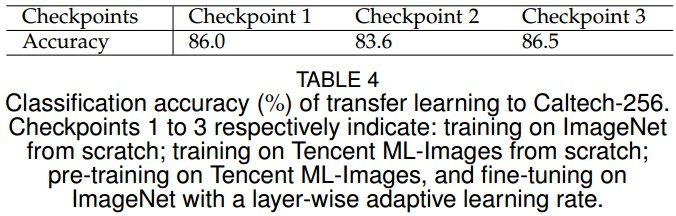### 4.3. 迁移学习到目标检测任务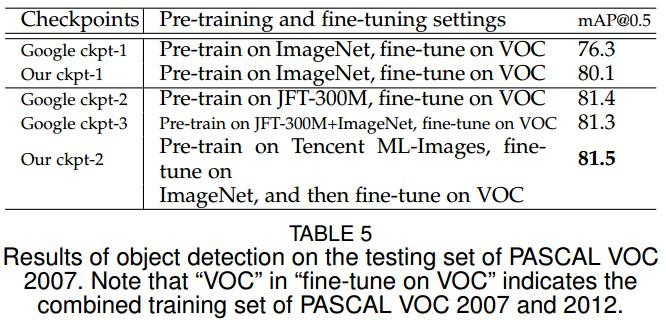### 4.4. 迁移学习到语义分割任务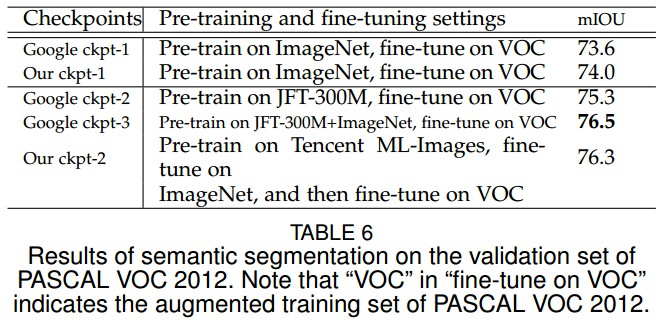Last modification：January 12th, 2019 at 10:35 am